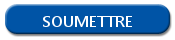# Format and data type in R

## Vectors

You can manually create vectors thanks to the c() function. Here are some examples:

```a <- c(1, 2, 3) # numerical vector
b <- c("one", "two", "three") # characters vector
c <- c(TRUE, FALSE, FALSE) # Boolean vector```

## Matrix

In R programming, matrix is kind of table that contain datasets. The main characteristic is the type of the data used on the table. Each column must have the same data type. The length of each column must be the same. Adding data on a matrix is done linearly, by line with the parameter byrow = TRUE or by column with the parameter byrow = FALSE. Here is an example of matrix creation.

```data <- c(98,901,33,27) # my data vector
rowNames <- c("Row1", "Row2") # a vector that contain the name of the lines
colNames <- c("Col1", "Col2")
myMatrix <- matrix(data, nrow=2, ncol=2, byrow=TRUE,
dimnames = list(rowNames, colNames))```

## Data frames

If matrix must have the same data type, this is not the case for data frames.

Data frames can have columns with different data types. So, you can associate columns with numbers, characters, Booleans…

To create a data frame, you can use the data.frame() function.

Here is an example:

```a <- c(1,2,3,4) # numerical vector
b <- c("red", "white", "red", NA) # character vector
c <- c(TRUE,TRUE,TRUE,FALSE) # Boolean vector
myDataframe <- data.frame(a,b,c) # my data frame```

## Lists

The lists are collections of ordered objects that can contain several types of data. To create a list, use  the list() function. Here is an example.

`myList <- list(myCharacters = "Emma", myVector = a, myMatrix = y, myNumber = 42, myBoolean = TRUE)`